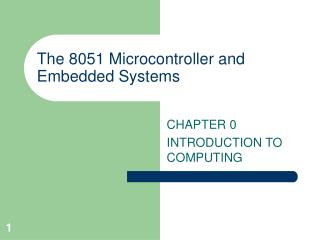DownloadDownload PresentationThe 8051 Microcontroller and Embedded Systems

# The 8051 Microcontroller and Embedded Systems

Download Presentation## The 8051 Microcontroller and Embedded Systems

- - - - - - - - - - - - - - - - - - - - - - - - - - - E N D - - - - - - - - - - - - - - - - - - - - - - - - - - -
##### Presentation Transcript

1. The 8051 Microcontroller and Embedded Systems CHAPTER 0 INTRODUCTION TO COMPUTING

2. Before you can drive the Robotic Car, you must pass your HW Code Test then your PDL.

3. OBJECTIVES • Convert any number from base 2, base 10, or base 16 to either of the other two bases • Add and subtract hex numbers • Add binary numbers • Represent any binary number in 2's complement • Represent an alphanumeric string in ASCII code • Describe logical operations AND, OR, NOT, XOR, NAND, NOR • Use logic gates to diagram simple circuits • Explain the difference between a bit, a nibble, a byte, and a word • Give precise mathematical definitions of the terms kilobyte, megabyte, gigabyte, and terabyte. • Explain the difference between RAM and ROM and describe their use • Describe the purpose of the major components of a computer system • List the three types of buses found in computers and describe the purpose of each type of bus • Describe the role of the CPU in computer systems • List the major components of CPU and describe the purpose of each

4. SECTION 0.2: DIGITAL PRIMER • Binary logic Figure 0–2Binary Signals

5. SECTION 0.2: DIGITAL PRIMER • Logic gates

6. SECTION 0.2: DIGITAL PRIMER • Logic design using gates Figure 0–3Two Implementations of a Half-Adder

7. SECTION 0.2: DIGITAL PRIMER Figure 0–4Block Diagram of a Half-Adder

9. SECTION 0.2: DIGITAL PRIMER Figure 0–6 3-Bit Adder Using 3 Full-Adders

10. SECTION 0.2: DIGITAL PRIMER Figure 0–7 Address Decoders

11. SECTION 0.2: DIGITAL PRIMER Figure 0–8 D Flip-Flops

12. SECTION 0.3: INSIDE THE COMPUTER • Some important terminology

13. SECTION 0.3: INSIDE THE COMPUTER • Internal organization of computers Figure 0–9 Inside the Computer

14. SECTION 0.3: INSIDE THE COMPUTER • More about the data bus

15. SECTION 0.3: INSIDE THE COMPUTER • More about the address bus Figure 0–10 Internal Organization of Computers

16. SECTION 0.3: INSIDE THE COMPUTER • Inside CPUs Figure 0–11 Internal Block Diagram of a CPU

17. SECTION 0.3: INSIDE THE COMPUTER • Internal working of computers

18. Next … • Lecture Problems Textbook Chapter 0 • Answer as many questions as you can and submit via MeL before the end of the lecture. (HINT: Do the easy questions first) • Proteus Exercise Textbook Chapter 0 • Do as much of the Proteus exercise as you can and submit via MeL before the end of the lecture.